##### Actions

To give a simpler proof of the Weierstrass approximation theorem (cf. Weierstrass theorem), S.N. Bernstein [a3] defined a new sequence of linear positive operators defined for functions continuous on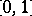as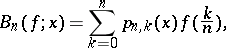whereThis sequence turned out to be a very interesting sequence, easy to deal with and having many applications in mathematical analysis. It has been extensively studied by G.G. Lorentz in [a13]. It is also of great interest in approximation theory, and as a result of that L.V. Kantorovich [a12] devised a modification of these operators, known as the Bernstein–Kantorovich polynomials; they are defined over a larger class of functions, e.g.,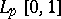,. The operator is defined as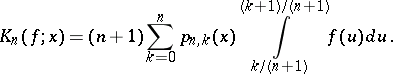The approximation properties of this sequence have been discussed in detail (cf., e.g., [a7], [a14]).

Another interesting modification of the Bernstein polynomials was introduced by J.L. Durrmeyer [a8] for approximating functions in,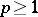. This sequence is given by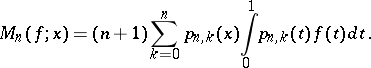M.M. Derriennic [a5] has studied approximation properties of the operators. Subsequently, important contributions to the study of these operators were made (see, e.g., [a6], [a9]). Modifications similar to that of Durrmeyer have been studied for other sequences of operators as well, e.g., for Szász, Lupas, and Baskakov operators (cf., e.g., [a10], [a11], [a17]).

It has been observed that the order of approximation by these sequences of linear positive operators increases with the smoothness of the function. E. Voronovskaya [a20] has shown that the order of approximation by Bernstein polynomials cannot be improved beyond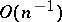. This phenomenon is true for every other sequence of linear operators. The first attempt at somehow improving the order of approximation was made by P.L. Butzer [a4], who showed that by taking a linear combination of the Bernstein polynomials the order of approximation considerably improves for smoother functions. More general combinations have been studied for other sequences of operators (cf., e.g., [a15]). There is still another approach towards improving the order of approximation, by taking iterative combinations (cf., e.g., [a16]).

Another phenomenon of interest is the study of simultaneous approximation (approximation of derivatives of a function by the derivatives of corresponding order of operators). The first remarkable result in this direction is due to Lorentz [a13]. For further research in this area, see [a5], [a6], [a9], [a11], [a17].

V.A. Baskakov [a1] gave a sequence of linear positive operators which includes the Bernstein polynomials, the Szász operators, the Lupas operators, etc., as particular cases. The sequence is defined by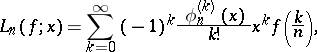whereis a family of real-valued functions such that:

i)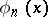can be expanded in a Taylor series in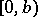(where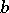may be equal to);

ii)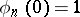;

iii)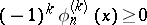,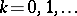, for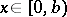;

iv),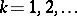,, for some constant;

v) for any fixed constant,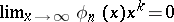for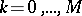. Studies similar to those for the Bernstein polynomials have been carried out for the Baskakov operators (cf., e.g., [a10], [a11], [a15]).

The converse problem of inferring the nature of smoothness of a function from its order of approximation led to the study of inverse and saturation theorems for the above sequences of operators. Such problems have been studied both locally as well as globally.

Some important references for the study of such problems are [a2], [a6], [a7], [a9], [a15], [a16], [a18], [a19].